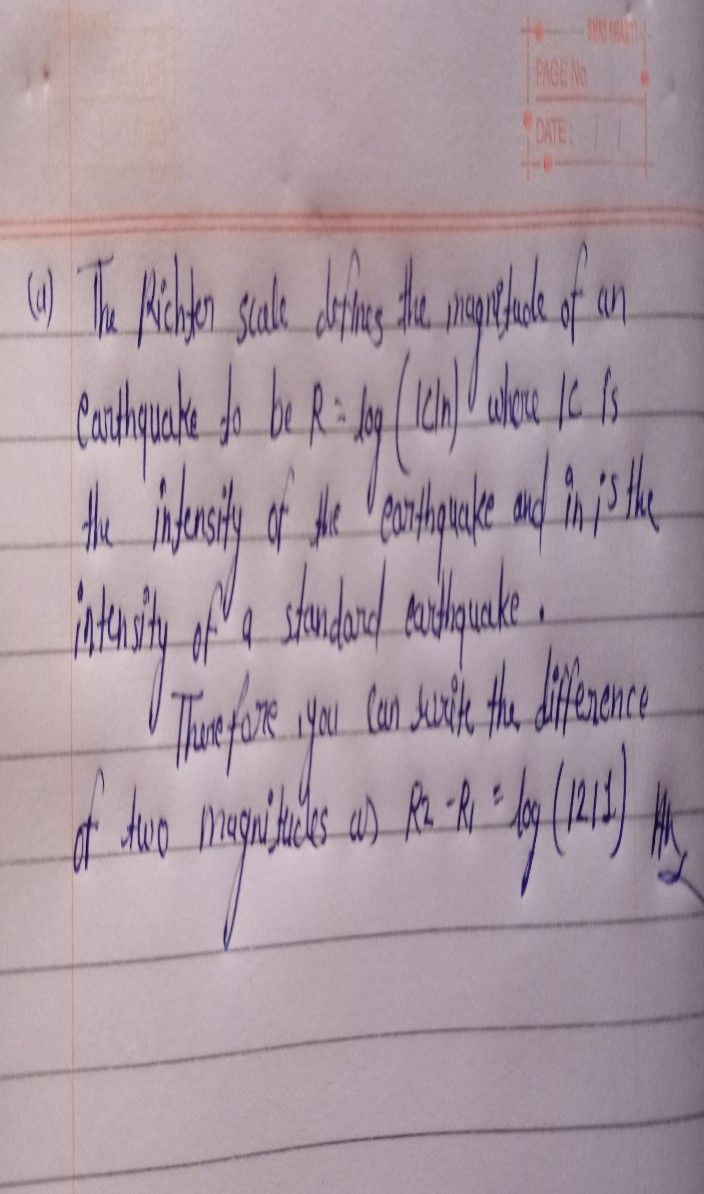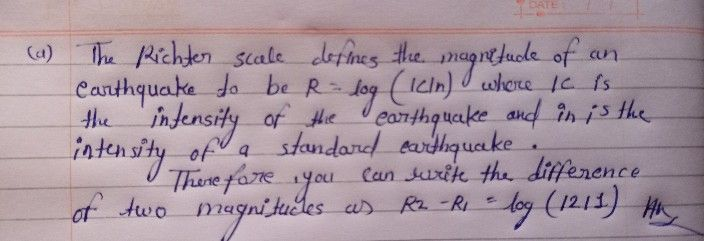Symbol
Problem$3$ Solve the following problems involving logarithmic functions. $1$ Suppose that the earthquake released approximately $10^{12}$ Joules of energy. /Formula: $R-23logk$ $o$ $\left(a$ What is its magnitude on a Richter scale? (b) How much more energy does this earthquake release than the reference earthquake?
10th-13th grade
Other
SolutionQanda teacher - missPreetiIf you have any doubts please do let me know dear. if you are satisfied with my answer please do LIKE and give a 5 STAR RATING and coins as THANK U GIFT..! ALL THE BEST..!!!If you have more questions then please ask through preffered match from my profile